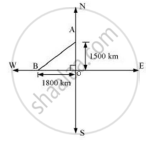Share

Books Shortlist
Your shortlist is empty

# Solution for An Aeroplane Leaves an Airport and Flies Due North at a Speed of 1000km/Hr. at the Same Time, Another Aeroplane Leaves the Same Airport and Flies Due West at a Speed of 1200 Km/Hr. How Far Apart Will Be the Two Planes After 1 Hours? - CBSE Class 10 - Mathematics

ConceptApplication of Pythagoras Theorem in Acute Angle and Obtuse Angle

#### Question

An aeroplane leaves an airport and flies due north at a speed of 1000km/hr. At the same time, another aeroplane leaves the same airport and flies due west at a speed of 1200 km/hr. How far apart will be the two planes after 1 hours?

#### SolutionDistance traveled by the plane flying towards north in 1 1/2hrs

= 1000xx1 1/2=1500km

Similarly, distance travelled by the plane flying towards west in 1 1/2hrs

=1200xx1 1/2=1800km

Let these distances are represented by OA and OB respectively.

Now applying Pythagoras theorem

Distance between these planes after 1 1/2hrs AB =sqrt(OA^2+OB^2)

=sqrt(1500^2+1800^2)

=sqrt(2250000+3240000)

=sqrt5490000

=sqrt(9xx610000)

=300sqrt61

So, distance between these planes will be 300sqrt61 km, after 1 1/2hrs

Is there an error in this question or solution?

#### Video TutorialsVIEW ALL 

Solution for question: An Aeroplane Leaves an Airport and Flies Due North at a Speed of 1000km/Hr. at the Same Time, Another Aeroplane Leaves the Same Airport and Flies Due West at a Speed of 1200 Km/Hr. How Far Apart Will Be the Two Planes After 1 Hours? concept: Application of Pythagoras Theorem in Acute Angle and Obtuse Angle. For the course CBSE
S